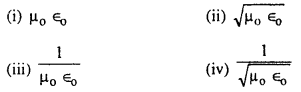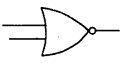# Bihar Board 12th Physics Objective Important Questions Part 4

BSEB Bihar Board 12th Physics Important Questions Objective Type Part 4 are the best resource for students which helps in revision.

## Bihar Board 12th Physics Objective Important Questions Part 4

Question 1.
Primary rainbow is formed due to:
(i) one reflection
(ii) one total internal reflection
(iii) one dispersion
(iv) all of these
(ii) one total internal reflection

Question 2.
Velocity of light is C in vacuum. In the glass (µ = 1.5) it will be:
(i) $$\frac{3}{2}$$C
(ii) $$\frac{2}{3}$$C
(iii) C
(iv) $$\frac{1}{2}$$C
(ii) $$\frac{2}{3}$$C

Question 3.
The velocity of light is maximum in:
(i) air
(ii) glass
(iii) water
(iv) vacuum
(iv) vacuum

Question 4.
The speed of em wave in vaccum is equal to:(iv) $$\frac{1}{\sqrt{\mu_{0} \epsilon_{0}}}$$

Question 5.
The velocity of light in a medium depends on:
(i) the intensity of the source
(ii) the frequency of the source
(iii) None of these
(ii) the frequency of the source

Question 6.
Nature of light waves is:
(i) transverse
(ii) longitudinal
(iii) some times longitudinal some times transverse
(iv) None of these
(i) transverse

Question 7.
Light waves are proved transverse by:
(i) interference
(ii) diffraction
(iii) polarisation
(iv) None
(iii) polarisation

Question 8.
The vlocityof light in galss, whose refractive index µg = 1.5 is 2 × 108m/s. In certain liquid the velocity of light is found to be 2.6 × 108 m/s. The refractive index (µc)of the liquid is.
(i) 1.5
(ii) 1.2
(iii) 1
(iv) 2.1
(ii) 1.2

Question 9.
Path difference in destructive interference is equal to:
(i) nλ
(ii) (n + 1)λ.
(iii) (2n + 1)λ
(iv) (2n + 1)$$\frac{\lambda}{2}$$
(iv) (2n + 1)$$\frac{\lambda}{2}$$

Question 10.
Brewster’s law is:
(i) µ = sin ip
(ii) µ = cos ip
(iii) µ = sin ip
(iv) µ = tan2 ip
(iii) µ = sin ip

Question 11.
Precious stones an detected with the help of
(i) ultraviolet rays
(ii) infrared rays
(iii) x-rays
(iv) None of the above
(i) ultraviolet rays

Question 12.
Laser beam is:
(i) highly monochromatic
(ii) monochromatic
(iii) heterogeneous in nature
(iv) monochromatic or not depends upon the energy available
(i) highly monochromatic

Question 13.
The laser is a source of:
(i) electric current
(ii) sound
(iii) incoherent light
(iv) coherent light
(iv) coherent light

Question 14.
Electromagnetic waves are:
(i) longitudinal
(ii) compressional
(iii) transverse
(iv) both transverse and longitudinal
(iii) transverse

Question 15.
X-rays or λ = 1A have freq. of:
(i) 3 × 108 Hz
(ii) 3 × 1018 Hz
(iii) 3 × 1010 Hz
(iv) 3 × 1015 Hz
(ii) 3 × 1018 Hz

Question 16.
Cathode rays are:
(i) electrons
(ii) neutrons
(iii) protons
(iv) photons
(i) electrons

Question 17.
The molecules of an atom consists of:
(i) protons
(ii) protons and electrons
(iii) protons and neutrons
(iv) α-particles
(iii) protons and neutrons

Question 18.
Electron volt is:
(i) unit of charge
(ii) unit of potential difference
(iii) unit of current
(iv) unit of energy
(iv) unit of energy

Question 19.
The charge of an electron is equal to:
(i) 1.6 × 10-1 C
(ii) 1.6 × 10-9 C
(iii) 1.6 × 10-19 C
(iv) 1.6 C
(iii) 1.6 × 10-19 C

Question 20.
The size of the nucleus is of the order of:
(i) 10-13 cm
(ii) 10-23 cm
(iii) 10-8 cm
(iv) 10-10 cm
(i) 10-13 cm

Question 21.
Which one of the following will deflect in electric field
(i) r-rays
(ii) x-rays
(iii) Uv rays
(iv) cathode rays
(iv) cathode rays

Question 22.
Work function is the energy required:
(i) to excite the atom
(ii) just to eject electron form the surface
(iii) to produce x-rays
(iv) None of the above
(ii) just to eject electron form the surface

Question 23.
Relation between mass and energy is:
(i) E = mc2
(ii) E = mc
(iii) E = mc3
(iv) None of these
(i) E = mc2

Question 24.
(ii) electron
(iii) proton
(iv) neutron
(iv) neutron

Question 25.
β-particles are fast moving:
(i) electrons
(ii) positrons
(iii) protons
(iv) neutrons
(i) electrons

Question 26.
γ-rays are similar to:
(i) α-rays
(ii) Cathode rays
(iii) X-rays
(iv) None of the above
(iii) X-rays

Question 27.
According to maxwell’s hypothesis, a changing electric field gives rise to:
(i) an emf
(ii) Electric current
(iv) magnetic field
(iv) magnetic field

Question 28.
The number of protons in an atom of atomic number Z and mass number A is:
(i) Z
(ii) A
(iii) A – Z
(iv) A + Z
(i) Z

Question 30.
Phase diff. between electric and magnetic field in e.m. wave is:
(i) Zero
(ii) 90°
(iii) 120°
(iv) 180°
(i) Zero

Question 31.
Which has higher frequency in e.m. spectrum?
(i) α-rays
(ii) β-rays
(iii) γ-rays
(iv) cathode rays
(iii) γ-rays

Question 32.
(i) fusion of nuclei
(ii) the decay of nuclei
(iii) the production of x-rays
(iv) thermionic emission
(ii) the decay of nuclei

Question 33.
If T is the temperature of the filament, the thermionic current varies as:
(i) 7
(ii) T1/2
(iii) T2
(iv) T3
(iii) T2

Question 34.
The energy of a photon of a wavelength is:
(i) $$\frac{h}{\lambda}$$
(ii) $$\frac{h}{c}$$
(iii) $$\frac{h c}{\lambda}$$
(iv) $$\frac{h \lambda}{c}$$
(iii) $$\frac{h c}{\lambda}$$

Question 35.
The angluar momentum of an electron of mass m, charge erevolving in a circular orbit is L then its mag.movent is
(i) eLm
(ii) $$\frac{e L}{m}$$
(iii) $$\frac{e L}{2 m}$$
(iv) $$\frac{2 e L}{m}$$
(iii) $$\frac{e L}{2 m}$$

Question 36.
The quantum of light is known as:
(i) photon
(ii) proton
(iii) hyperon
(iv) phonon
(i) photon

Question 37.
A thermionic diode is used as:
(i) an amplifier
(ii) an oscillator
(iii) a modulator
(iv) a rectifier
(iv) a rectifier

Question 38.
The action of a radio value is based on the principle of:
(i) thermionic emission
(ii) induction
(iii) Lenz’s law
(iv) Ohm’s law
(i) thermionic emission

Question 39.
P-N Junction is used for:
(i) the measurement of temperature
(ii) converting A.C. into D.C.
(iii) obtaining higher voltage from low voltage
(iv) amplifying feeble currents.
(ii) converting A.C. into D.C.

Question 40.
The number of photons of freq. 1014 Hz in radiation of 6.62 J will be
(i) 1010
(ii) 1015
(iii) 1020
(iv) 1025
(iii) 1020

Question 41.
According to J.C.Bose, the range of wave length in e.m. wave is:
(i) 1 mm -10 mm
(ii) 25 mm – 50 mm
(iii) 200 mm – 500 mm
(iv) None of these
(ii) 25 mm – 50 mm

Question 42.
Ozone layer is situated from the surface of earth:
(i) 40 km – 50 km
(ii) 100 km – 300 km
(iii) 500 km ~ 1000 km
(iv) None of them
(i) 40 km – 50 km

Question 43.
Ionosphere is situated from the surface of earth:
(i) 50 km – 80 km
(ii) 200 km – 400 km
(iii) 500 km – 1000 km
(iv) None of them
(ii) 200 km – 400 km

Question 44.
Boolean expression for NOR gate is
(i) $$\overline{A \cdot B}$$ = y
(ii) $$\overline{A+B}$$ = y
(iii) A·B = y
(iv) A + B = y
(ii) $$\overline{A+B}$$ = y

Question 45.
The logic symbolstands for
(i) OR-Gate
(ii) AND gate
(iii) NOR gate
(iv) NAND gate
(iii) NOR gate

Question 46.
The height of TV transmission tower is 245 m at a place on the earth. The distance upto which the broadcasting can be send from the tower as-
(ii) 245 m
(i) 245 km
(iii) 50 km
(iv) 112 km
(iii) 50 km

Question 47.
For TV Broadcast which type of modulation is used for audio signals?
(i) Amplitude modulation
(ii) Pulse modulation
(iii) Freq. modulation
(iv) None of these
(iii) Freq. modulation

Column-I – Column-II
1. William Gilbert → Electricity
2. Coulomb → Force between to electric charges
3. Karl F. Gauss → Gauss theorem
4. Benjamin Franklin → Kite experiment
5. Ven de Graff → Generator
6. Michael Faraday → Discovery of e.m. induction Law of electrolysis
→ Relation between light and magnetism
7. Joule → Law of heating effect
8. Tolman and Stewart → Experiment on Free electron of charge carriers
9. George simon ohm → Ohm’s law or Relation between current of p.d
10. Gustav Robert Kirchoff → Junction rule or Loop rule
11. Sir Charles F. Wheat stone → Thermo electricity
12. Thomas Johann seebeck → Thermo electricity
13. J.C.A Petties → Thermo electricity
14. Hans Christian Orested → Connection between electricity Magnetism
15. Jeans-Baptise Biot + Felix savart → Measurement of magnetic field due to current
16. Andre marie Ampere → unit of electric current
17. Fleming → Left hand rule and Diode valve
18. ‘D’Arsonoval → Galvanometer
19. Lawrence → Cyclotron
20. Nicola Tesla → Tesla (unit of magnetic field), ganerator or dynamo
21. Lenz → The direction of induced e.m.f. & current
22. Maxwell → Electrommagnetic wave
23. Hertz → Electron magnetic wave + unit of frequency
24. Christain Huygen → wave theory of light
25. Snell → Law of refraction
26. Galileo → Telescope
27. Newton → Telescope, surpascular theory of light
28. Thomas young → Interference of light
29. Fresnel → Diffraction of light
30. Fraunhofer → Diffraction of light & Dark line in visual spectrum
31. Willian Nicol → Nicol prism for producing plane polarised light
32. Sir David Brewster → Brewster’s law of relation between µ and ip
33. Rontgen → X-rays
34. J.J. Thomson → Electron, nature of cathode rays, mass spectrograph & experiment on electron diffraction
35. Ernest Rutherford → Nucleus, proton and Scattering of α – particle
36. Neil Bohr → Atom model, hydrogen atom.
37. Einstein → Photo electric equation, Theory of relativity.
38. Hallwachs → Photo electric effect.
39. Leonard → Law of photo electric effect.
40. Millikan → Law of photo electric effect.
41. Max Planck → Quantum theory of radiation.
42. Sir William Bragg → Wave length of X-rays
43. Sir Lawrence Bragg → Wave length of X-rays
45. Heisenberg → Composition of Nuclei
46. Henry Becquerel → Radio activity